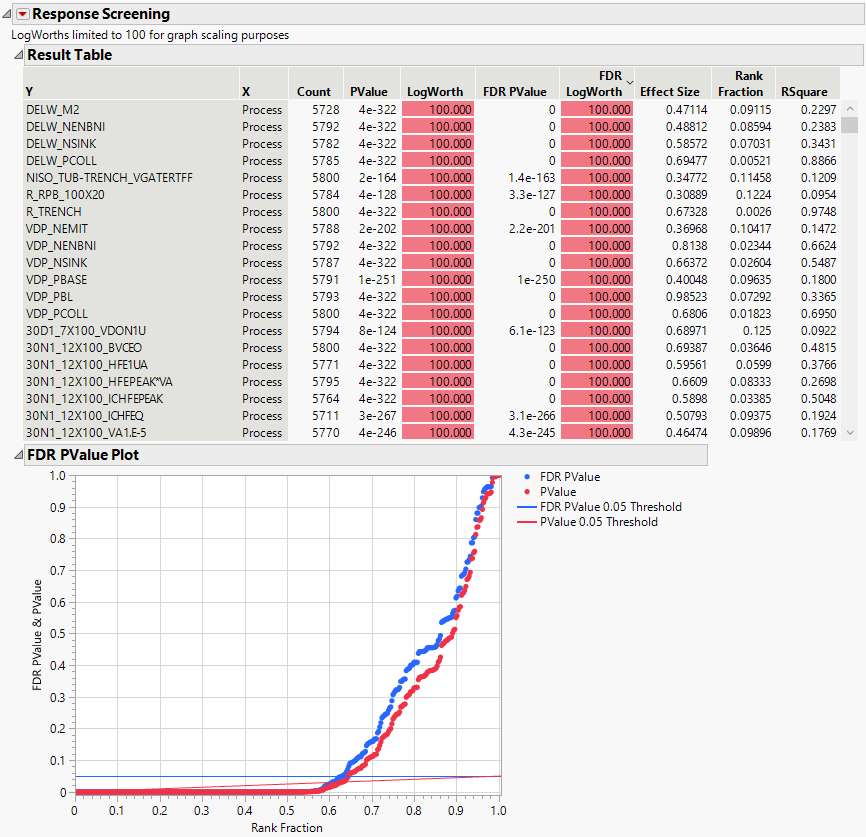Predictive and Specialized Modeling > Response Screening > Example of Response Screening
Publication date: 05/24/2021

# Example of Response Screening

The Probe.jmp sample data table contains 387 characteristics (the Responses column group) measured on 5800 wafers. The Lot ID and Wafer Number columns uniquely identify the wafer. You are interested in which of the characteristics show different values across a process change (Process).

1. Select Help > Sample Data Library and open Probe.jmp.

2. Select Analyze > Screening > Response Screening.

3. Select the Responses column group and click Y, Response.

4. Select Process and click X.

5. Enter 100 in the MaxLogWorth box.

A logworth (-log10(p-value)) of 100 or larger corresponds to an extremely small p-value. Setting a value for the MaxLogWorth helps control the scale of plots as it limits the reported logworth value.

6. Click OK.

The report shows a table of p-value results and the FDR PValue Plot, and also contains two other plot reports.

The FDR PValue Plot shows two types of p-values, FDR PValue and PValue, for each of the 387 tests. These are plotted against Rank Fraction. PValue is the usual p-value for the test of a Y against Process. The FDR PValue is a p-value that is adjusted to guarantee a given false discover rate (FDR), here 0.05. The FDR PValues are plotted in blue and the PValues are plotted in red. The Rank Fraction ranks the FDR p-values from smallest to largest, in order of decreasing significance.

Both the horizontal blue line and the sloped red line on the plot are thresholds for FDR significance. Tests with FDR p-values that fall below the blue line are significant at the 0.05 level when adjusted for the false discovery rate. Tests with ordinary p-values that fall below the red line are significant at the 0.05 level. In this way, the plot enables you to read FDR significance from either set of p-values.

Figure 22.2 Response Screening Report for 387 Tests against ProcessThe FDR PValue Plot shows that more than 60% of the tests are significant. A handful of tests are significant using the unadjusted p-value, but not significant using the FDR p-value. These tests correspond to the red points that are above the red line, but below the blue line.

To identify the characteristics that are significantly different across Process, you can drag a rectangle around the appropriate points in the plot. This selects the rows corresponding to these points in the Result table, where the names of the characteristics are given in the first column.

The Result table contains 387 rows, one for each response measure in the Responses group. The response is given in the first column, called Y. Each response is tested against the effect in the X column, namely, Process.

The remaining columns give information about the test of Y against X. Here the test is a Oneway Analysis of Variance. In addition to other information, the table gives the test’s p-value, LogWorth, FDR (False Discovery Rate) p-value, and FDR LogWorth. Use this table to sort by the various statistics, select rows, or plot quantities of interest.

Notice that LogWorth and FDR LogWorth values that correspond to p-values of 1e-100 or less are reported as 100, because you set MaxLogWorth to 100 in the launch window. Also, cells corresponding to FDR LogWorth values greater than two are colored with an intensity gradient.